$$\require{cancel}$$

# 2.7: Example Problems

## 2.7.1 Plane Symmetry.

### (1) A Uniformly Charged Plane.

Consider a plane which is infinite in extent and uniformly charged with a density of σ Coulombs/m2 ; the normal to the plane lies in the z-direction, Figure (2.7.6). The charge plane is located at z=0. It is clear from symmetry that the electric field can have only a component normal to the plane since for every charge element at x,y there is an exactly similar charge element at -x,-y such that the transverse field components at the point of observation cancel. All of the charges on a ring of radius r produce the same z-component of electric field at the point of observation, P; they may therefore be lumped together to obtain

$\left.d E_{p}\right|_{z}=\frac{d q}{4 \pi \epsilon_{0}} \frac{\cos (\theta)}{R^{2}}=\frac{\sigma 2 \pi r d r}{4 \pi \epsilon_{0}} \frac{z}{R^{3}}, \nonumber$

therefore

$E_{z}=\left(\frac{\sigma}{2 \epsilon_{0}}\right) z \int_{0}^{\infty} \frac{r d r}{\left(z^{2}+r^{2}\right)^{3 / 2}}. \nonumber$

Upon carrying out the integration one obtains an electric field that is independent of z and, for positive z, has the value $$\sigma / 2 \epsilon_{0}$$: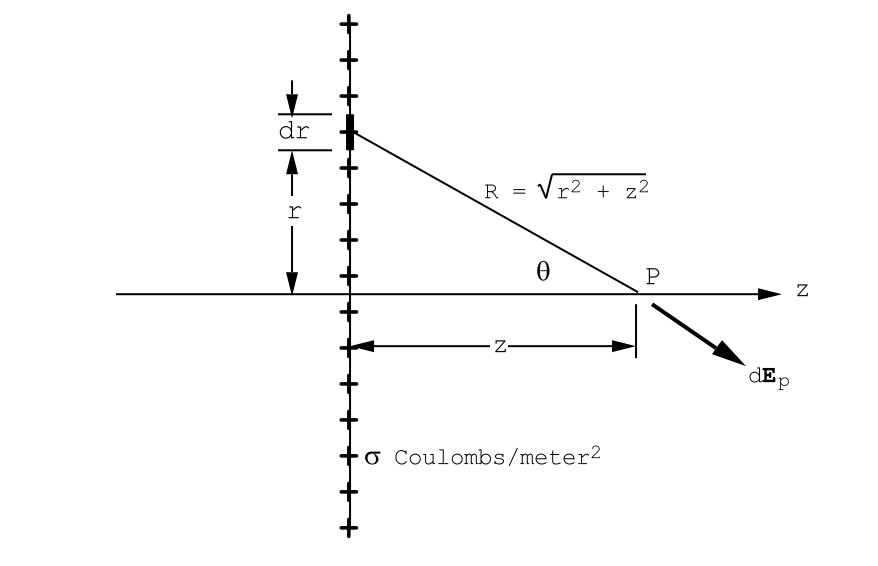Figure $$\PageIndex{6}$$: Calculation of the electric field generated by a uniformly charged plane.

$E_{z}=\frac{\sigma}{2 \epsilon_{0}}. \label{2.25}$

Note that for points to the left of the charge plane the electric field points along -z: ie. $$E_{z}=-\sigma / 2 \epsilon_{0}$$. There is a discontinuity of magnitude $$\sigma / \epsilon_{0}$$ in the z-component of the electric field at the charge plane.

### (2) The Potential Function for a Uniformly Charged Plane.

It is interesting to calculate the potential function from a direct application of Equation (2.2.4) for the potential generated by a charge distribution. Referring to Figure (2.7.6) one can calculate the contribution to the potential at the observation point P:

$d V_{p}=\frac{d q}{4 \pi \epsilon_{0}} \frac{1}{R}=\frac{\sigma 2 \pi r d r}{4 \pi \epsilon_{0}} \frac{1}{\sqrt{r^{2}+z^{2}}}\nonumber$

and therefore for z>0

$V_{p}=\frac{\sigma}{2 \epsilon_{0}} \int_{0}^{\infty} \frac{r d r}{\sqrt{r^{2}+z^{2}}}=\frac{\sigma}{2 \epsilon_{0}}[\sqrt{\infty}-z]. \nonumber$

This is an example of an integration that does not converge. In order to obtain a proper integral one can use a trick. Suppose that the charged plane was not infinite in extent, but that it was in the form of a large, but finite, disc having a radius D. There would then be no problem; one would have

$V_{p}=\frac{D \sigma}{2 \epsilon_{0}}-\frac{z \sigma}{2 \epsilon_{0}}=\text { constant }-\frac{z \sigma}{2 \epsilon_{0}}.\nonumber$

One is always free to subtract a constant from the potential without changing the value of the corresponding electric field distribution. One can simply subtract the constant from the above equation to obtain

$V_{p}=-\frac{z \sigma}{2 \epsilon_{0}}, \quad \text { for } z>0. \nonumber$

Notice that Vp does not change sign for z less than zero because the integration involves the square root of z2 . Thus the complete expression for the potential function, valid for all values of z, is given by

$V_{p}=-\frac{\sigma}{2 \epsilon_{0}}|z|, \label{2.26}$

see Figure (2.7.7). In Equation (\ref{2.26}) the zero for the potential function has been chosen so that the potential is zero on the plane. The potential function is continuous as the field point P moves through the plane from left to right in Figure (2.7.7): the electric field component normal to the plane undergoes a discontinuity.

### (3) The Field of a Uniformly Charged Plane Using Gauss’ Theorem.

We could have deduced that $$\vec E$$ is independent of position, except at the charge plane, directly from the fourth Maxwell equation $$\operatorname{div}(\overrightarrow{\mathrm{E}})=\left(1 / \epsilon_{0}\right)\left(\rho_{f}-\operatorname{div}(\overrightarrow{\mathrm{P}})\right)$$. In this application there is no electric dipole density since $$\vec P$$ = 0 everywhere. Consequently, also $$\operatorname{div}(\overrightarrow{\mathrm{P}})=0$$ everywhere.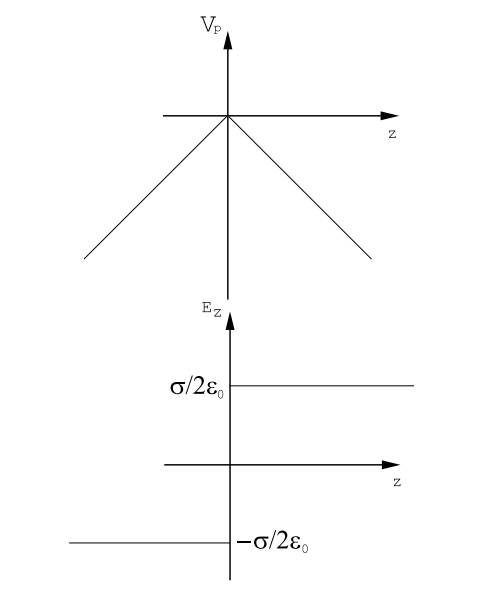Figure $$\PageIndex{7}$$: The potential function (top) and the electric field (bottom) generated by a uniformly charged infinite plane carrying a charge density of σ Coulombs/m2. $$E_{z}=-\frac{\partial V_{p}}{\partial z}.$$

As we have seen in (1) above using symmetry arguments $$\vec E$$ has only a z-component; moreover, this component, Ez, cannot depend upon x,y if the charge distribution is uniform and infinite in extent: any shift of the infinite charge distribution in the x-y plane can not be detected by a fixed observer. Therefore, in this case,

$\operatorname{div}(\overrightarrow{\mathrm{E}})=\frac{\partial E_{z}}{\partial z}=0 \nonumber$

except at z=0 since the charge density is zero everywhere except at z=0. Thus Ez = constant everywhere except at z=0.Figure $$\PageIndex{8}$$: Geometry for the application of Gauss’ Theorem to calculate the electric field strength generated by an infinite, plane, uniformly charged sheet whose density is σ Coulombs/m2 . The magnitude of the resulting field is $$E_{0}=\sigma /\left(2 \epsilon_{0}\right)$$.

Moreover, it is obvious from Coulomb’s law and symmetry that the magnitude of the electric field for z < 0 must have the same value as the magnitude of the electric field for z > 0, although the direction of the field switches 180 degrees upon passage through the charge plane. For this very symmetric case one can obtain the magnitude of the electric field directly from Gauss’ Theorem.

$\int \int \int_{V} d V d i v(\overrightarrow{\mathrm{E}})=\int \int_{S} d S(\overrightarrow{\mathrm{E}} \cdot \hat{\mathbf{n}})=\frac{Q}{\epsilon_{0}}, \nonumber$

where V is the volume bounded by the closed surface S and Q is the total charge contained in the volume. Apply this theorem to a parallelepiped having its edges oriented along the co-ordinate axes as shown in Figure (2.7.8). The box shown in the figure contains an amount of charge σAz Coulombs since the area of the side of the box whose normal lies along z is just Az. Now calculate the surface integral of the electric field over the surface of the

parallelepiped. This integral is easy to carry out because the electric field is constant in magnitude and in direction. Over four sides of the box shown in Figure (2.7.8) the direction of the electric field is parallel with the surface, thus perpendicular to the surface normals, and the scalar product of the electric field and the normal to the surface, $$\hat{\mathbf{n}}$$, vanishes so that these sides contribute nothing to the surface integral. Over the two ends of the box the electric field is parallel with the surface normal so that the scalar product $$\vec E$$· $$\hat{\mathbf{n}}$$ just becomes an ordinary product, and the contribution to the surface integral from each end is E0Az: notice that at each end the electric field is parallel with the direction of the outward normal because the field reverses direction from one side of the charge sheet to the other. The surface integral in Gauss’ theorem is given by

$\int \int_{S} d S(\overrightarrow{\mathrm{E}} \cdot \hat{\mathbf{n}})=2 E_{0} A_{z}. \nonumber$

But the total charge contained in the box is σAz, so that the electric field must have the magnitude $$E_{0}=\sigma /\left(2 \epsilon_{0}\right)$$ in agreement with Equation (\ref{2.25}).

### (4) An Electric Double Layer.

Consider two oppositely charged uniform charge sheets separated by a distance of 2d meters, as illustrated in Figure (2.7.9). The electric field generated by each charge sheet is uniform, independent of z, and directed normal to the planes of charge. In the regions outside the charged planes,ie. z > d or z < d, the electric field is zero because the fields generated by the oppositely charged planes have opposite directions and therefore cancel. Between the two charged planes the fields due to the two planes have the same orientation and therefore add to produce the total field $$E_{z}=-\sigma / \epsilon_{0}$$. A plot of Ez vs z exhibits discontinuities at z= d, Figure (2.7.10). The potential function outside the double layer is constant corresponding to zero electric field. However, the potential on the right hand side of the double layer is different from the potential on the left hand side. The step in the potential is related to the strength of the double layer:

$\Delta V=\frac{2 \sigma d}{\epsilon_{0}}=P_{d} / \epsilon_{0}, \nonumber$

where Pd is the dipole moment per unit area in Coulombs/meter.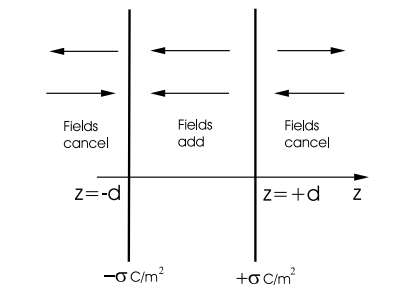Figure $$\PageIndex{9}$$: An electric double layer consists of two infinite plane sheets of charge densities +σ and −σ Coulombs/m2 separated by a small distance. The electric field is zero everywhere outside the double layer, but is equal to $$\left|E_{z}\right|=\sigma / \epsilon_{0}$$ between the two charged sheets.

### (5) A Uniformly Polarized Slab.

Consider a slab that is uniformly polarized along the z-axis as shown in Figure (2.7.11). The strength of the polarization density is P0, and there are no free charges anywhere. One can define a bound charge density from the relation ρb = −div($$\vec P$$). This bound charge density generates an electric field just as surely as does the free charge density, ρf . Inside the slab of Figure (2.7.11) the polarization density is $$\overrightarrow{\mathrm{P}}=\mathrm{P}_{0} \hat{\mathbf{u}}_{z}$$; thus ∂Pz/∂z is zero inside the slab and therefore div($$\vec P$$), and hence ρb, is zero. Outside the slab $$\vec P$$ = 0 so that here also div($$\vec P$$) = 0 and therefore ρb = 0. One might be misled by the fact that the bound charge density vanishes both inside and outside the slab into thinking that the bound charge density is zero everywhere. However, the bound charge density does not vanish on the slab surfaces. The derivative ∂Pz/∂z is singular at z=0 and at z=d ie. on the faces of the slab. This singularity is integrable: if one integrates the derivative from z = −$$\epsilon$$ toFigure $$\PageIndex{10}$$: The electric field distribution and the corresponding potential function generated by an electrical double layer. The electric field intensity inside the double layer is $$E_{0}=-\sigma / \epsilon_{0}$$. The jump in the potential across the double layer is $$\Delta V=2 \sigma d / \epsilon_{0}$$.Figure $$\PageIndex{11}$$: A uniformly polarized slab. The polarization density,$$\vec P$$0, is directed along the normal to the slab. The discontinuities in the normal component of the polarization produce effective surface bound charge densities given by $$\sigma_{b}=-\operatorname{div}(\overrightarrow{\mathrm{P}})$$.

z = +$$\epsilon$$, for example, the result is

$\int_{-\epsilon}^{+\epsilon}\left(\frac{\partial \mathrm{P}_{z}}{\partial z}\right) d z=\mathrm{P}_{z}(+\epsilon)-\mathrm{P}_{z}(-\epsilon)=\mathrm{P}_{0}, \nonumber$

because Pz(−$$\epsilon$$) = 0 and Pz(+$$\epsilon$$) = P0. Notice that the value of the integral is independent of the small interval ǫ, and, in particular, it remains finite even in the limit as $$\epsilon$$ → 0. The integrand has the character of a Dirac δ − function: $$\partial \mathrm{P}_{z} / \partial z=\mathrm{P}_{0} \delta(z)$$, where the function δ(z) is a strongly peaked function having zero width but infinite amplitude in such a way that it’s integral is just equal to unity. But this means that the charge density on the surface at z=0, ρb = −∂Pz/∂z, z, is a very sharply peaked integrable function of z: it is in fact a surface charge density of strength −P0 Coulombs/meter2 . Similarly, there will be a surface charge density of strength +P0 Coulombs/meter2 on the surface at z=d. The electric field distribution produced by a uniformly polarized slab in which the polarization density lies parallel with the slab normal is exactly the same as that which would be produced by two uniformly charged planes carrying charge densities of σ = ±P0, see Figure (2.7.9). Outside the uniformly polarized slab the electric field is zero. Inside the slab there is a uniform electric field, $$\mathrm{E}_{z}=-\mathrm{P}_{0} / \epsilon_{0}$$, whose direction is opposite to the direction of the polarization density; it is called a depolarizing field because it tends to act so as to reduce the polarization density. The potential outside the slab will be constant, both on the left and on the right, but the potential on the right will be larger than that on the left by the amount $$\Delta V=\mathrm{P}_{0} d / \epsilon_{0}$$ Volts. It is interesting to examine the auxiliary field $$\overrightarrow{\mathrm{D}}=\epsilon_{0} \overrightarrow{\mathrm{E}}+\overrightarrow{\mathrm{P}}$$. Outside the slab both $$\vec E$$ and $$\vec P$$ are zero so that $$\vec D$$ must also be zero. Inside the slab $$\vec D$$ has only a z-component, and

$\mathrm{D}_{z}=\epsilon_{0} \mathrm{E}_{z}+\mathrm{P}_{z}=-\mathrm{P}_{0}+\mathrm{P}_{0} \equiv 0. \nonumber$

The normal component of $$\vec D$$ is continuous across the slab surfaces. In general, in the absence of any surface free charge density the normal component of $$\vec D$$ must be continuous across the interface.

Now consider a uniformly polarized slab in which the polarization density vector lies in the plane of the slab as shown in Figure (2.7.12). In this case the polarization has only an x-component, and that x-component, Px, is a function only of z. This means that $$\operatorname{div}(\overrightarrow{\mathrm{P}})=\partial \mathrm{P}_{x} / \partial x=0$$ everywhere. There is no bound charge density anywhere, and there is no free charge density, by supposition, so that there are no sources for the electric field. A uniformly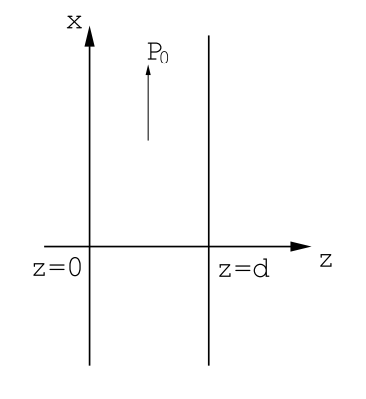Figure $$\PageIndex{12}$$: An infinite slab that is uniformly polarized in plane, Px = P0.

polarized slab in which the polarization lies in the slab plane generates no macroscopic electric field.

It is a general rule that a spatial variation of the polarization density, $$\vec P$$, produces an effective volume charge density $$\rho_{b}=-\operatorname{div}(\overrightarrow{\mathrm{P}})$$. In addition to this effective volume charge density, any discontinuity in the normal component of $$\vec P$$ across a surface produces an effective surface charge density given by

$\sigma_{b}=-\left(\mathrm{P}_{2 n}-\mathrm{P}_{1 n}\right). \nonumber$

These bound volume and surface charge densities must be used, along with the free charge distributions, to calculate the electric field and potential distributions.

### 2.7.2 A Spherically Symmetric Charge Distribution.

Consider a charge distribution that is spherically symmetric but one in which the charge distribution ρ(r) may have an arbitrary dependence upon the co-ordinate r, see Figure (2.7.13). In this case the electric field can have only a radial component by symmetry. The magnitude of this radial component, Er, cannot depend on position on the surface of a spherical surface centered onFigure $$\PageIndex{13}$$: The electric field intensity generated by a spherically symmetric charge distribution. The electric field has only a radial component because the transverse components generated by two equivalent charges, dq1 and dq2, cancel by symmetry.

the center of symmetry of the charges because any rotation of the distribution around the center of symmetry leaves the charge distribution unaltered. It follows that the surface integral of the electric field over the surface of a sphere of radius R is just $$4 \pi R^{2} \mathrm{E}_{r}) so that from Gauss’ theorem $\mathrm{E}_{r}=\frac{1}{4 \pi \epsilon_{0}}\left(\frac{1}{R^{2}}\right) \int_{0}^{R} d r \rho(r) 4 \pi r^{2}. \nonumber$ Thus for the electric field outside the charge distribution one has $4 \pi R^{2} \mathrm{E}_{r}=\frac{1}{\epsilon_{0}} \int_{S p h e r e} \rho(r) d V o l=\frac{Q}{\epsilon_{0}}. \label{2.27}$ Here Q is the total charge contained in the distribution. The electric field outside a spherically symmetric charge distribution looks like the field of a point charge! The potential function generated by any symmetric charge distribution must be independent of the spherical polar co-ordinates θ, φ because Eθ and Eφ are both zero. The potential function can be calculated from ∂V/∂r = −Er. Note that the potential must be a continuous function of r even if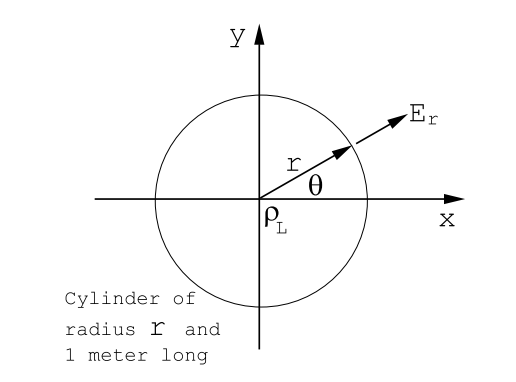Figure \(\PageIndex{14}$$: The electric field generated by a uniformly charged line lying along the z-axis. The line charge density is ρL Coulombs/meter. The electric field is radial and has the value $$\mathbf{E}_{r}=\left(\rho_{L} / 2 \pi \epsilon_{0}\right)(1 / r)$$ Volts/m.

the charge density distribution, ρ(r), contains discontinuities. The potential function is continuous everywhere except on a surface containing an electrical double layer.

## 2.7.3 Cylindrical Symmetry.

### (1) A uniformly Charged Line.

Let charges be distributed uniformly along the z-axis with a charge density of ρL Coulombs/meter as shown in Figure (2.7.14). It is easy to see, using Coulomb’s law, that the electric field generated by this distribution can have only a radial component; the transverse components generated by equivalent charge elements symmetrically disposed around the origin at +z and at -z cancel each other out. Furthermore, the radial electric field strength,Er, cannot depend upon the angle θ because the line charge exhibits rotational symmetry; ie. the charge distribution remains unaltered by a rotation through any angle around the z-axis. Er also cannot depend upon position along z since the line is taken to be infinitely long. Apply Gauss’ theorem to a cylindrical surface centered on the line charge, and 1 meter long and having a radius of r meters. The charge contained within this cylinder is ρL Coulombs. The surface integral of the electric field is easy to carry out because the electric field is parallel with the surface normal at every point on the cylinder surface; on the end surfaces the electric field contributes nothing to the surface integral because it lies in the surface, i.e. $$\overrightarrow{\mathrm{E}} \cdot \hat{\mathbf{u}}_{n}=0$$, where $$\hat{\mathbf{u}}_{n}$$ is the unit normal to the surface. It follows from Gauss’ Theorem that

$2 \pi r \mathrm{E}_{r}=\frac{\rho_{L}}{\epsilon_{0}}, \nonumber$

and therefore

$\mathrm{E}_{r}=\frac{\rho_{L}}{2 \pi \epsilon_{0}}\left(\frac{1}{r}\right) \quad \operatorname{Volts} / m. \nonumber$

Since the electric field has only a radial component it follows that the corresponding potential function, V, cannot depend upon the co-ordinates z,θ. But Er = −∂V/∂r, so that one can use

$\mathrm{V}(r)=-\left(\frac{\rho_{L}}{2 \pi \epsilon_{0}}\right) \ln (r)\nonumber$

as the potential function for a uniformly charged line. The logarithmic variation of V(r) with r can also be deduced from a direct application of Poisson’s equation, Equation (2.2.5). In this application the free charge density ρf = 0 everywhere except at the origin, r=0. There is assumed to be no electric dipole distribution anywhere so that $$\vec P$$ = 0 and ρb = −div($$\vec P$$) = 0 everywhere. Thus ∇2V = 0. But in cylindrical polar co-ordinates, and in the absence of any variation of V with angle or with displacement along z

$\nabla^{2} \mathrm{V}=\frac{\partial}{\partial r}\left(r \frac{\partial \mathrm{V}}{\partial r}\right)=0. \nonumber$

It follows from this that r∂V/∂r = constant = a, and therefore V = a ln(r) + constant in agreement with the above expression for V(r): remember that one can add or subtract a constant from the potential function without changing the electric field calculated from it.

### (2) A Line of Dipoles.

Consider a line of dipoles uniformly distributed along the z-axis with a density of PL Coulombs; the dipoles are supposed to be oriented along the x-directionFigure $$\PageIndex{15}$$: An infinite line of uniformly distributed point dipoles can be modelled by a uniformly charged positive line separated by a small distance d from a uniformly charged negative line. Let the charge density on the positive line be ρL, and let the charge density on the negative line be −ρL, then the dipole density, a vector, is given by $$\left|\overrightarrow{\mathrm{P}}_{L}\right|=\rho_{L} d$$ Coulombs, and $$\vec P$$L is directed from the negative line to the positive line.

as shown in Figure (2.7.15). The line of dipoles can be modelled by two line charges separated by a very small distance d, as shown in Figure (2.7.15). The resulting potential function at P, the point of observation, written in cylindrical polar co-ordinates can be calculated as follows using the potential function for a uniformly charged line:

$\mathrm{V}_{P}=-\frac{\rho_{L}}{2 \pi \epsilon_{0}} \ln \left(r_{+}\right)+\frac{\rho_{L}}{2 \pi \epsilon_{0}} \ln \left(r_{-}\right)=\frac{\rho_{L}}{2 \pi \epsilon_{0}} \ln \left(r_{-} / r_{+}\right). \nonumber$

But $$r_{-} \cong r_{+}+d \cos (\theta)$$ so that

$\mathrm{V}_{P}=\frac{\rho_{L}}{2 \pi \epsilon_{0}} \ln \left(1+\frac{d \cos (\theta)}{r_{+}}\right)\nonumber$

and

$\lim _{d \rightarrow 0} \mathrm{V}_{P}=\frac{\rho_{L} d}{2 \pi \epsilon_{0}} \frac{\cos (\theta)}{r}=\frac{1}{2 \pi \epsilon_{0}} \frac{\overrightarrow{\mathrm{P}}_{L} \cdot \vec{r}}{r^{2}}, \nonumber$

where PL is the line density of dipoles. The electric field components are

$\mathrm{E}_{r}=-\frac{\partial \mathrm{V}_{P}}{\partial r}=\frac{\mathrm{P}_{L}}{2 \pi \epsilon_{0}} \frac{\cos (\theta)}{r^{2}},\nonumber$

$\mathrm{E}_{\theta}=-\frac{1}{r} \frac{\partial \mathrm{V}_{P}}{\partial \theta}=\frac{\mathrm{P}_{L}}{2 \pi \epsilon_{0}} \frac{\sin (\theta)}{r^{2}},\nonumber$

$\mathrm{E}_{z}=0. \nonumber$

A cross-section through the electric field distribution in a plane normal to the line of dipoles looks similar to the field distribution around a point dipole. The electric field at θ = 0 is directed along the +x direction and so is the field at θ = $$\pi$$: both fields have the strength $$\mathrm{P}_{L} /\left(2 \pi \epsilon_{0} r^{2}\right)$$. The electric fields at θ = $$\pi$$/2, 3$$\pi$$/2 are directed along the -x direction, and have the strength $$\mathrm{E}_{\theta}=\mathrm{P}_{L} /\left(2 \pi \epsilon_{0} r^{2}\right)$$.

### 2.7.4 A Uniformly Polarized Ellipsoidal Body.Figure $$\PageIndex{16}$$: A uniformly polarized body having an irregular shape. The resulting surface bound charge distribution produces an electric field distribution that is non-uniform both inside and outside the body.

Consider a uniformly polarized body of arbitrary shape that is immersed in vacuum. The bound volume charge density associated with a uniform polarization density is zero since div($$\vec P$$) = 0. The bound surface charge density is not zero because there is a discontinuity in the normal component of $$\vec P$$ on the surface of the body; this surface charge density is given by $$\sigma_{b}=\overrightarrow{\mathrm{P}}_{0} \cdot \hat{\mathbf{n}}$$ where $$\hat{\mathbf{n}}$$ is the unit vector normal to the surface of the body. Notice that this surface charge density varies from place to place on the surface and that it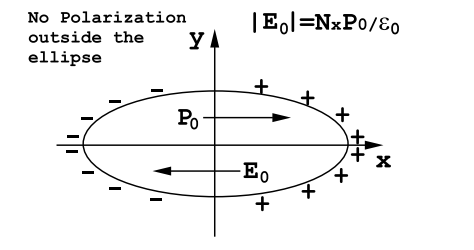Figure $$\PageIndex{17}$$: A uniformly polarized ellipsoidal body; the polarization lies along a principle axis of the ellipse. The resulting surface bound charge distribution produces a uniform electric field inside the ellipse. The electric field distribution outside the ellipse is non-uniform.

changes sign when one considers opposite points on the surface, see Figures (2.7.16 and 2.7.17). In fact, it is a consequence of charge conservation that the sum over all surface charges on a body that carries no free charges must be zero. The surface bound charge density distribution can be used to calculate the electric field and potential function everywhere in space. An analytical calculation is usually out of the question, and the problem must usually be solved by means of a numerical summation. One could, in principle, calculate the electric field components by means of Coulomb’s law, but it is usually more convenient to work with the integral for the potential function, Equation (2.2.6). For the particular case in which the uniformly polarized body has an ellipsoidal shape the calculation of the potential function and the electric field can be carried out analytically. See, for example, J.A. Stratton, Electromagnetic Theory, McGraw-Hill, N.Y., 1941, section 3.25. The surprising result is that the electric field inside a uniformly polarized ellipsoid is uniform. Usually this internal electric field is not parallel with the direction of the polarization density. The internal electric field and the polarization are parallel only if the polarization vector is directed along a principal axis of the ellipsoid defined by the equation for its surface

$\left(\frac{x}{a}\right)^{2}+\left(\frac{y}{b}\right)^{2}+\left(\frac{z}{c}\right)^{2}=1. \label{2.28}$

Here a,b,c are the three semi-axes that define the ellipsoid. Outside the ellipsoid the electric field is not uniform: it resembles a distorted dipole field and falls off at large distances like 1/r3 . In the principle axis co-ordinate system defined by Equation (\ref{2.28}) the electric field components inside the ellipsoid are simply related to the polarization components:

$\mathrm{E}_{x} =-\mathrm{N}_{x} \frac{\mathrm{P}_{x}}{\epsilon_{0}}, \label{2.29}$
$\mathrm{E}_{y} =-\mathrm{N}_{y} \frac{\mathrm{P}_{y}}{\epsilon_{0}}, \nonumber$
$\mathrm{E}_{z} =-\mathrm{N}_{z} \frac{\mathrm{P}_{z}}{\epsilon_{0}}. \nonumber$

The depolarization coefficients Nx, Ny, and Nz are pure numbers that depend upon the parameters of the ellipsoid (a,b,c of Equation (\ref{2.28})). They can be calculated using elliptic integrals. The depolarization coefficients obey a sum rule:

$\mathrm{N}_{x}+\mathrm{N}_{y}+\mathrm{N}_{z}=1. \label{2.30}$

This sum rule makes it very easy to deduce values for the depolarization coefficients for very symmetrical bodies. Consider the following examples:

(a) Sphere. By symmetry Nx = Ny = Nz. Therefore from the sum rule each must be equal to 1/3

(b) Cylinder. This is the limiting case in which one dimension of the ellipsoid, the z dimension say, becomes very long. The two transverse depolarizing factors must be equal by symmetry. On the other hand, if the cylinder is polarized along its length any surface bound charge density can only be associated with the ends; but the ends are infinitely far away and consequently any charges on them produce an infinitely small electric field. This means that a cylinder that is uniformly polarized along its length will produce no electric field. It can be concluded that for such a cylinder Nz = 0. It follows from the sum rule that if Nx = Ny then each must be equal to 1/2.

(c) A Thin Flat Disc. Think of an ellipsoid of revolution which is thin along the z-direction but which has a very large radius R in the xy plane. By symmetry Nx = Ny . If the edges of the disc are very far from its center, ie. R → ∞, then the electric field near the center of the disc due to surface charges on the disc edges must become vanishingly small ( remember thatFigure $$\PageIndex{18}$$: Oblate ellipsoid of revolution.Figure $$\PageIndex{19}$$: Cigar shaped ellipsoid of revolution.

the field due to an element of charge decreases like 1/R2 ). It follows that Nx = Ny → 0 in the limit as R → ∞. From the sum rule Equation (\ref{2.30}) one can conclude that Nz → 1. The field inside an infinite disc that is polarized parallel with its axis of symmetry has the value $$\mathrm{E}_{z}=-\mathrm{P}_{z} / \epsilon_{0}$$ in agreement with the result previously deduced for a transversely polarized slab, section (5) above, and Figure (2.7.11).

Two commonly encountered special cases of ellipsoids of revolution are shown in Figures (2.7.18 and 2.7.19). In each case one need only specify the depolarizing coefficient for the axis of revolution, the z-axis. The other two depolarizing factors are equal and can be calculated from the sum rule Equation (\ref{2.30}). Case(a) is a pancake shaped ellipsoid, Figure (2.7.18). For this case

$\mathrm{N}_{z}=\frac{\mathrm{R}^{2} \mathrm{d}}{\left(\mathrm{R}^{2}-\mathrm{d}^{2}\right)^{3 / 2}}\left(\frac{\sqrt{\mathrm{R}^{2}-\mathrm{d}^{2}}}{\mathrm{d}}-\arctan \left(\frac{\sqrt{\mathrm{R}^{2}-\mathrm{d}^{2}}}{\mathrm{d}}\right)\right), \quad \text { where }(\mathrm{d} / \mathrm{R})<1. \label{2.31}$

In the limit d/R → 0 the depolarizing factor is given approximately by

$\mathrm{N}_{z} \cong 1-\frac{\pi}{2}\left(\frac{\mathrm{d}}{\mathrm{R}}\right). \label{2.32}$

Case(b) is a cigar shaped ellipsoid, Figure (2.7.19). For this case

$\mathrm{N}_{z}=\frac{\left(1-\psi^{2}\right)}{\psi^{3}}\left(\frac{1}{2} \ln \left(\frac{1+\psi}{1-\psi}\right)-\psi\right), \text { where } \psi=\sqrt{1-(\mathrm{R} / \mathrm{d})^{2}}. \label{2.33}$

In the limit as the cigar becomes very long, (d/R) → ∞ the demagnetizing coefficient can be expressed as

$\mathrm{N}_{z} \cong\left(\frac{\mathrm{R}}{\mathrm{d}}\right)^{2}\left(\ln \left[\frac{2 \mathrm{d}}{\mathrm{R}}\right]-1\right).\label{2.34}$

For a general ellipsoid it can be shown that

$\begin{array}{l} \mathrm{N}_{x}=\left(\frac{a b c}{2}\right) \int_{0}^{\infty} \frac{d s}{\left(s+a^{2}\right) R_{s}} \\ \mathrm{N}_{y}=\left(\frac{a b c}{2}\right) \int_{0}^{\infty} \frac{d s}{\left(s+b^{2}\right) R_{s}} \\ \mathrm{N}_{z}=\left(\frac{a b c}{2}\right) \int_{0}^{\infty} \frac{d s}{\left(s+c^{2}\right) R_{s}} \end{array}$

where $$R_{s}=\left(\left[s+a^{2}\right]\left[s+b^{2}\right]\left[s+c^{2}\right]\right)^{1 / 2}$$.

(See J.A. Stratton, Electromagnetic Theory, McGraw-Hill, N.Y., 1941. Section 3.27.)